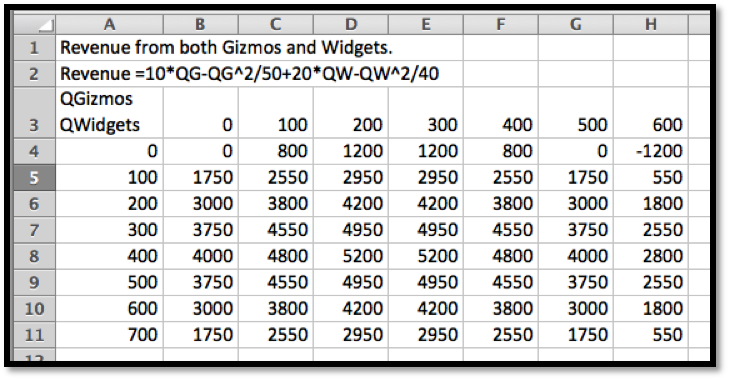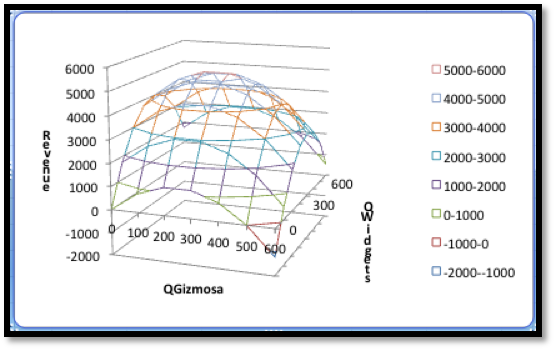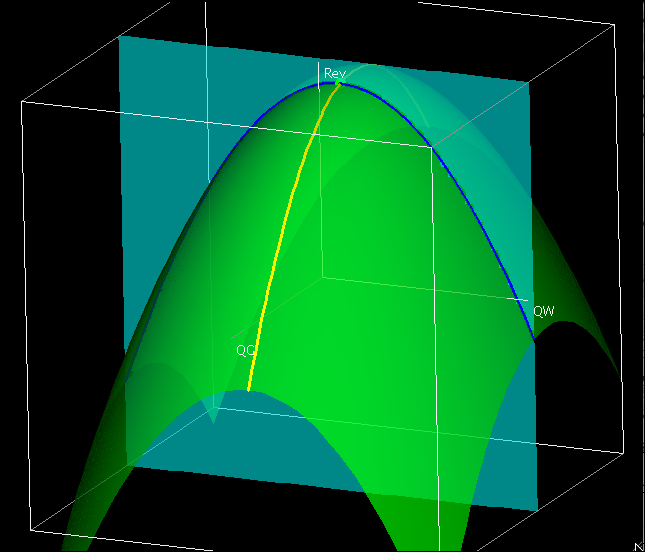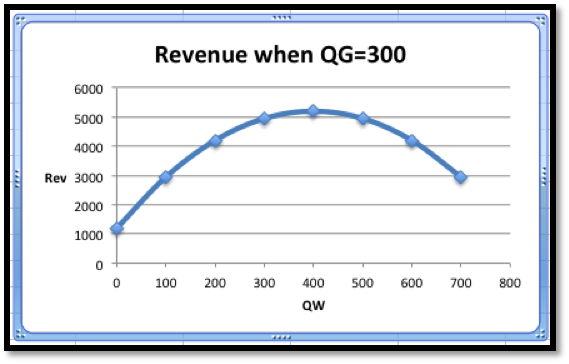## Section6.2Wire Frames, Partial Derivatives, and Tangent Planes

A standard technique in mathematics courses is to try to break a complicated problem into smaller and easier problems. For functions of several variables this can be done by looking at the variables one at a time, and treating the other variables as constants. Then we are back to considering functions of a single variable.

### Subsection6.2.1Wire frames

We start by returning to Example 6.1.12 from Section 6.1, and seeing what information can be obtained by looking at one variable at a time.

#### Example6.2.1.Optimizing Revenue with Two Products.

I have a company that produces two products, widgets and gizmos. The two demand functions are:
\begin{align*} \PriceGizmo\amp =10-\frac{\QuantityGizmo}{50}=10-\frac{\text{QG}}{50}\\ \PriceWidget\amp =20-\frac{\QuantityWidget}{40}=20-\frac{\text{QW}}{40}\text{.} \end{align*}
This gives me the following revenue function:
\begin{equation*} \revenue(\QG,\QW)=10QG-\frac{\QG^2}{50}+20\QW-\frac{\QW^2}{40}\text{.} \end{equation*}
Look at the functions of one variable obtained by treating either QG or QW as a constant. Use this information to find where we maximize revenue.
Solution.
In terms of the last example, we want to start with a table and a wire frame chart.The wires are obtained by intersecting the graph of the function with a plane where QW or QG is held constant.Thus, when we treat either QW or QG as a constant we effectively are looking at one of the wires of the wire frame. To illustrate this, we will look at the wires corresponding to $$\QW=400$$ and $$\QG=300\text{.}$$ When $$\QG=300\text{,}$$ our revenue function simplifies to
\begin{align*} \revenue(300,\QW) \amp =3000-1800+20\text{QW}-\frac{\text{QW}^2}{40}\\ \amp =1200+20\QW-\frac{\QW^2}{40}\text{.} \end{align*}
Thus, the wire corresponding to $$\QG=300$$ is a parabola that bends down.
The interactive below shows how the wireframe is built from cuves defined by slice curves defined by cut planes.To find the vertex of the parabola, we take the derivative of our function of QW and set it equal to zero.
\begin{equation*} \frac{d}{d\QW} \revenue(300,\QW)=20-\frac{\QW}{20}\text{.} \end{equation*}
This derivative is zero when $$\QW=400\text{.}$$ That is the only possible place on this wire where we can have a maximum.
Similarly, when $$\QW=400\text{,}$$
\begin{align*} \revenue(\QG,400) \amp =10\QG-\frac{\QG^2}{50}+4000\\ \frac{d}{d\QG} \revenue(\QG,400)\amp =10-\frac{\QG}{25}\text{.} \end{align*}
This derivative is zero when $$\QG=250\text{.}$$ That is the only possible place on this wire where we can have a maximum.
##### 7.
The function is the Cobb-Douglas production function in a country,
\begin{equation*} \Production(\Labor,\Capital)=10 \Labor^{0.74}\Capital^{0.26}\text{,} \end{equation*}
where labor is in millions of workers, capital equipment is in units of billions of dollars, and production is in units of billions of dollars per year. In the $$(\Labor,\Capital)$$ plane, let $$P_1=(300,30)\text{,}$$ and $$P_2=(310,32)\text{.}$$
Solution.
1. Give the 2 functions of one variable through $$P_1$$ obtained by holding each variable constant.
\begin{align*} \Production(300,C) \amp = 10*300^{0.74} C^{0.26}=680.879 C^{0.26} \\ \Production(L,30) \amp = 10 L^{0.74} 30^{0.26}=24.2132 L^{0.74} \text{.} \end{align*}
Hence both cross sections are exponential function.
2. Find the partial derivatives of the original function.
\begin{align*} \Production_L (L,C)\amp =\frac{\partial}{\partial L} 10 L^{0.74} C^{0.26}\\ \amp \text{(Take the partial derivative wrt }L\text{; Treat }C\text{ as a constant.)}\\ \amp =10 (0.74) L^{-0.26} C^{0.26}\text{.} \end{align*}
Then we have
\begin{align*} \Production_L (L,C) \amp = \frac{7.4 C^{0.26}}{L^{0.26}}\\ \Production_C (L,C) \amp = \frac{\partial}{\partial C} 10 L^{0.74} C^{0.26}\\ \amp \text{(Take the partial derivative wrt }C\text{; Treat }L\text{ as a constant.)}\\ \amp =10 L^{0.74} (0.26) C^{-0.74}\text{.} \end{align*}
Then we have that
\begin{equation*} \Production_C (L,C)=2.6 \frac{L^{0.74}}{C^{0.74}}\text{.} \end{equation*}
3. Evaluate the partial derivatives at $$P_1\text{.}$$
\begin{align*} \Production_L (300, 30)\amp =\frac{7.4 30^{0.26}}{300^{0.26}} \approx 4.07\\ \Production_C (300, 30)\amp =2.6 \frac{300^{0.74}}{30^{0.74}} \approx 14.29\text{.} \end{align*}
4. Give the equation of the tangent plane through $$P_1\text{.}$$
\begin{align*} \Production(300,30)\amp =10*30^{0.74} 30^{0.26}\approx 1648.62\\ \Linear \Production(L,C) \amp = Prod_L (L_0,C_0 )(L-L_0 )+Prod_C (L_0,C_0 )(C-C_0 )+Prod(L_0,C_0 )\\ \amp = 4.07(L-300)+14.29(C-30)+1648.62\text{.} \end{align*}
5. The approximation at $$P_2$$ obtained from the tangent plane.
\begin{equation*} \Linear \Production(310, 32) =4.07(310-300)+14.29(32-30)+1648.62=1717.9\text{.} \end{equation*}
So as both $$L$$ and $$C$$ increase, so does the production. Specifically increasing $$L$$ by 10 million workers and $$C$$ by 2 billion dollars would give an estimated increase in production of $$1717.9-1648.62=69.28$$ billions of dollars worth of units.

#### Exercise Group.

For the given functions and points $$P_1$$ and $$P_2\text{:}$$
1. Give the 3 functions of one variable through $$P_1$$ obtained by holding each variable constant.
2. Find the partial derivatives of the original function.
3. Evaluate the partial derivatives at $$P_1\text{.}$$
4. Give the equation of the linear approximating function through $$P_1\text{.}$$
5. The approximation at $$P_2$$ obtained from the function in d.
##### 8.
The function is $$f(x,y,z)=x^2+3xy+4y^2+2z^2+5xz\text{,}$$ $$P_1=(4,2,1)\text{,}$$ and $$P_2=(3,2.5,2)\text{.}$$
##### 9.
The function is $$f(x,y,z)=(x+3y-2z)/(x^2+y^2+z^2 )\text{,}$$ $$P_1=(2,3,-1)\text{,}$$ and $$P_2=(3,2.5,0)\text{.}$$
Solution.
1. Give the 3 functions of one variable through $$P_1$$ obtained by holding each variable constant.
\begin{align*} f(x,3,-1) \amp = \frac{x+9+2}{x^2+9+1}=\frac{x+11}{x^2+10}\\ f(2,y,-1) \amp = \frac{2+3y+2}{4+y^2+1}=\frac{3y+4}{y^2+5}\\ f(2,3,z) \amp =\frac{2+9-2z}{4+9+z^2 }=\frac{11-2z}{13+z^2 }\text{.} \end{align*}
2. Find the partial derivatives of the original function.
\begin{align*} f_x (x,y,z) \amp = \frac{(1)(x^2+y^2+z^2 )-(x+3y-2z)(2x)}{(x^2+y^2+z^2 )^2} \\ \amp = \frac{(x^2+y^2+z^2 )-(2x^2+6xy-4xz)}{(x^2+y^2+z^2 )^2 } \\ \amp =\frac{(-x^2+y^2+z^2-6xy+4xz)}{(x^2+y^2+z^2 )^2} \\ f_y (x,y,z) \amp = \frac{(3)(x^2+y^2+z^2 )-(x+3y-2z)(2y)}{(x^2+y^2+z^2 )^2 } \\ \amp = \frac{(3x^2+3y^2+3z^2 )-(2xy+6y^2-4yz)}{(x^2+y^2+z^2 )^2} \\ \amp =\frac{3x^2-3y^2+3z^2-2xy+4yz}{(x^2+y^2+z^2 )^2} \\ f_z (x,y,z) \amp = \frac{(-2)(x^2+y^2+z^2 )-(x+3y-2z)(2z)}{(x^2+y^2+z^2 )^2}\\ \amp = \frac{(-2x^2-2y^2-2z^2 )-(2xz+6yz-4z^2 )}{(x^2+y^2+z^2 )^2} \\ \amp =\frac{-2x^2-2y^2+2z^2-2xz-6yz}{(x^2+y^2+z^2 )^2} \text{.} \end{align*}
3. Evaluate the partial derivatives at $$P_1\text{.}$$
\begin{align*} f_x (2,3,-1) \amp = \frac{(-4+9+1-36-8)}{(4+9+1)^2} = -\frac{38}{196}= -\frac{19}{98}\\ f_y (2,3,-1) \amp = \frac{12-27+3-12-12}{196} =-\frac{-36}{196} =-\frac{-9}{49}\\ f_z (2,3,-1)\amp =\frac{-8-18+2+4+18}{196}= -\frac{2}{196}=-\frac{1}{98}\text{.} \end{align*}
4. Give the equation of the linear approximating function through $$P_1\text{.}$$
\begin{align*} \Linear f(x,y,z)\amp = f(2,3,-1)+f_x (2,3,-1) (x-2)+f_y (2,3,-1)(y-3)+f_z (2,3,-1)(z+1)\\ \amp = \frac{13}{14}-\frac{19}{98} (x-2)-\frac{9}{49} (y-3)-\frac{1}{98}(z+1)\text{.} \end{align*}
5. The approximation at $$P_2$$ obtained from the function in d.
\begin{align*} \Linear f(3,2.5,0)\amp = \frac{13}{14}-\frac{19}{98} (1)-\frac{9}{49} \left(-\frac{1}{2}\right)-\frac{1}{98} (1)\\ \amp =\frac{13*7}{14*7}+\frac{-19+9-1}{98}=\frac{91-11}{98}=\frac{80}{98}=\frac{40}{49}\text{.} \end{align*}
##### 10.
The function is $$f(x,y,z)=(x^2 z)(x+2^y+z^3)$$ $$P_1=(3,-1,1)\text{,}$$ and $$P_2=(2,0,1)\text{.}$$
##### 11.
The function is the revenue function for selling widgets, gizmos, and gadgets with demand price functions
and in $$(\QuantityGizmo,\QuantityWidget,\QuantityGadget)$$ space, $$P_1=(1000,500,700)\text{,}$$ and $$P_2=(1050,575,625)\text{.}$$
Solution.
We can do the entire problem in terms of gadgets, gizmos and widgets, but for notation sake we will replace them (alphabetically) as follows:
We need the revenue function and we have the price functions, so we have that
\begin{align*} \revenue \amp =R(x,y,z)= x \price_x+y \price_y+z \price_z\\ \amp =x \left(40-\frac{z}{45}-\frac{y}{600}-\frac{x}{300}\right)+y\left(30-\frac{y}{50}-\frac{z}{300}-\frac{x}{500}\right)\\ \amp \quad +z\left(20-\frac{z}{40}-\frac{y}{500}-\frac{x}{400}\right)\\ \amp = -\frac{x^2}{300}-\frac{11 x y}{3000}-\frac{89 x z}{3600}+40 x-\frac{y^2}{50}-\frac{2 y z}{375}+30 y-\frac{z^2}{40}+20 z\text{.} \end{align*}
(Note: the last step/simplification was done in Wolfram Alpha)
1. Give the 3 functions of one variable through $$P_1$$ obtained by holding each variable constant.
\begin{align*} R(x,500,700) \amp = \frac{1}{900} (-3 x^2+18775 x+8895000)\\ R(1000,y,700) \amp = \frac{1}{450} (-9 y^2+10170 y+9500000)\\ R(1000,500,z) \amp = \frac{1}{360} (-9 z^2-2660 z+16140000)\text{,} \end{align*}
so the cross sectional function are all quadratic (parabolas) that open downwards.
2. Find the partial derivatives of the original function.
Finding the partial derivatives when dealing with three variables is very similar to the procedure we use when we have two variables. We treat the other variables as constants.
\begin{align*} R_x (x,y,z) \amp = -\frac{x}{150}-\frac{11 y}{3000}-\frac{89 z}{3600}+40 \\ R_y (x,y,z) \amp = -\frac{11 x }{3000}-\frac{y}{25}-\frac{2 z}{375}+30 \\ R_z (x,y,z) \amp = -\frac{89 x }{3600}-\frac{2 y}{375}-\frac{z}{20}+20 \text{.} \end{align*}
3. Evaluate the partial derivatives at $$P_1\text{.}$$
\begin{align*} R_x (1000,500,700) \amp = -\frac{1000}{150}-\frac{5500}{3000}-\frac{89 (700)}{3600}+40=\frac{511}{36}\\ R_y (1000,500,700) \amp = -\frac{11000 }{3000}-\frac{500}{25}-\frac{1400}{375}+30=\frac{13}{5}\\ R_z (1000,500,700) \amp = -\frac{89000 }{3600}-\frac{1000 }{375}-\frac{700}{20}+20=-\frac{76}{18}\text{.} \end{align*}
4. Give the equation of the linear approximating function through $$P_1\text{.}$$
\begin{equation*} R(1000,500,700)=\frac{246700}{9}\text{.} \end{equation*}
Then the linear approximation is given by
\begin{align*} \Linear\revenue(x,y,z) \amp = R(1000,500,700)\\ \amp+R_x (1000,500,700) (x-1000)\\ \amp + R_y (1000,500,700)(y-500)\\ \amp+ R_z (1000,500,700)(z-700) \\ \amp = \frac{246700}{9}+\frac{511}{36} (x-1000)+\frac{13}{5} (y-500)\\ \amp-\frac{763}{18}(z-700)\text{.} \end{align*}
5. The approximation at $$P_2$$ obtained from the function in d.
\begin{align*} \Linear\revenue(1050,575,625) = \frac{246700}{9}+\frac{511}{36} (50)\\ \amp +\frac{13}{5} (75)-\frac{763}{18} (-75)=31,495\text{.} \end{align*}
mathstat.slu.edu/~may/ExcelCalculus/external/Examples/Section-6-2-Examples.xlsx